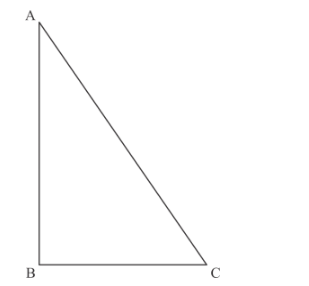# A right angled triangle whose sides are 3 cm, 4 cm and 5 cm

Question:

A right angled triangle whose sides are 3 cm, 4 cm and 5 cm is revolved about the sides containing the right angle in two ways. Find the difference in volumes of the two cones so formed. Also, find their curved surfaces.

Solution:

We consider the following figure as followsLet the angle B is right angle and the sides of the triangle are AB = 4cm, BC = 3cm,
AC = 5cm.

When the triangle is revolved about the side AB, then the base-radius, height and slant height of the produced cone becomes BCAB and AC respectively. Therefore, the volume of the produced cone is

$\mathrm{V}_{1}=\frac{1}{3} \pi \times \mathrm{BC}^{2} \times \mathrm{AB}$

$=\frac{1}{3} \pi \times(3)^{2} \times 4$

$=12 \pi$ cubic cm

In this case, the curved surface area of the cone is

$S_{1}=\pi \times \mathrm{BC} \times \mathrm{AC}$

$=\pi \times 3 \times 5$

$=15 \pi$ square $\mathrm{cm}$

When the triangle is revolved about the side BC, then the base-radius, height and slant height of the produced cone becomes ABBC and AC respectively. Therefore, the volume of the produced cone is

$V_{2}=\frac{1}{3} \pi \times \mathrm{AB}^{2} \times \mathrm{BC}$

$=\frac{1}{3} \pi \times(4)^{2} \times 3$

$=16 \pi$ cubic cm

In this case, the curved surface area of the cone is

$S_{2}=\pi \times \mathrm{AB} \times \mathrm{AC}$

$=\pi \times 4 \times 5$

$=20 \pi$ square $\mathrm{cm}$

Therefore, the difference between the volumes of the two cones so formed is

$V_{2}-V_{1}=16 \pi-12 \pi$

$=4 \pi \mathrm{cm}^{3}$

Hence the difference between the volumes is $4 \pi \mathrm{cm}^{3}$

And surface areas are $15 \pi \mathrm{cm}^{2}$ and $20 \pi \mathrm{cm}^{2}$